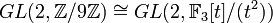# Isomorphic general linear groups not implies isomorphic rings

## Statement

### Statement for$n = 2$

It is possible to have two commutative unital rings$R_1,R_2$ such that the general linear group of degree two$GL(2,R_1)$ is isomorphic to the general linear group of degree two$GL(2,R_2)$, but$R_1$ and$R_2$ are not isomorphic as rings.

Two notable examples are:

•$GL(2,\mathbb{Z}/4\mathbb{Z}) \cong GL(2,\mathbb{F}_2[t]/(t^2))$: See general linear group:GL(2,Z4).
•$GL(2,\mathbb{Z}/9\mathbb{Z}) \cong GL(2,\mathbb{F}_3[t]/(t^2))$: See general linear group:GL(2,Z9).

(These are the only examples for finite discrete valuation rings, it seems).